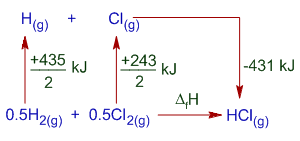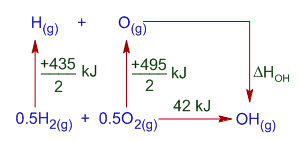< Previous question Next question >

# BOND DISSOCIATION ENTHALPY (BOND ENERGY) MCQ IIT JEE

## The bond dissociation enthalpy of gaseous H2, Cl2 and HCl are 435, 243 and 431 kJ mol-1, respectively. Calculate the enthalpy of formation of HCl gas.

(IIT JEE 1985)

Logic & Solution:

Bond dissociation enthalpy is defined as the amount of heat absorbed when one mole of specific bonds are cleaved homolytically in the gaseous state.

Note: There are other terms like bond enthalpy or bond energy or mean bond enthalpy, used through out the literature. These terms represent the average of bond dissociation enthalpies of same type of bonds present in molecules.

The thermochemical equations for bond dissociation of H2, Cl2 and HCl can be written as follows.

(1) H2(g) --------> 2H(g) ; ΔH1 = +435 kJ

(2) Cl2(g) --------> 2Cl(g) ; ΔH2 = +243 kJ

(3) HCl(g) --------> H(g) + Cl(g) ; ΔH3 = +431 kJ

Thermochemical equation for the formation of HCl is:

0.5H2(g) + 0.5Cl2(g) --------> HCl(g) ; ΔfH = ?

Above equation can be obtained by subtracting equation (3) from the sum of (1)/2+(2)/2

i.e., (1)/2 + (2)/2 - (1)

Therefore the ΔfH for HCl gas = (ΔH1/2)+(ΔH2/2)-ΔH3 = (435/2) + (243/2) - (431) = -92 kJ

Alternatively you can logically solve the problem as follows:

The formation of HCl from H2 and Cl2 occurs in the following steps:

i) the bonds between H-H and Cl-Cl are broken that require energy

ii) formation of new bond between H and Cl, which involve liberation of heat.Hence, according to Hess's law, the sum of enthalpy changes in above steps will give the enthalpy change for formation of HCl from H2 and Cl2.

i.e.,

ΔfH = (435/2) + (243/2) + (-431) = -92 kJ

Note: -431 kJ is the negative of bond dissociation enthalpy as shown in above diagram, since heat is liberated during the formation of H-Cl bond. It is reverse of bond dissociation of HCl and hence is exothermic.

## Related questions

### 0.5H2(g) + 0.5O2(g) ------> OH(g) ; ΔH = +42 kJ

H2(g) --------> 2H(g) ; ΔH = +435 kJ

O2(g) --------> 2O(g) ; ΔH = +495 kJ

(IIT JEE-1981)

Solution:

The thermochemical equation for bond energy or bond formation enthalpy for O-H radical can be written as follows:

O(g) + H(g) -------> OH(g)

We can get this equation as follows:

[0.5H2(g) + 0.5O2(g) ------> OH(g)] - 0.5[H2(g) --------> 2H(g)] - 0.5[O2(g) --------> 2O(g)]

Therefore:

bond energy =  +42 - 0.5(435) - 0.5(495) = -423 kJ

Alternatively, the bond energy can be calculated as follows:

O(g) + H(g) -------> OH(g) is the last step involved in the formation of OH from H2 and O2 as shown below.According to Hess's law: The enthalpy change is same whether the reaction occurs in one step or in several steps. i.e. the enthalpy change in direct step is equal to the sum of enthalpy changes in indirect method.

435/2 kJ + 495/2 kJ + ΔHOH = 42 kJ

or

ΔHOH = 42 kJ - 435/2 kJ - 495/2 kJ = -423 kJ

## Homework

1) What is enthalpy?

2) Why the enthalpy change in one reaction path is equal to that in another path?

 < Previous question Next question >

Author: Aditya vardhan Vutturi